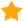2 doctors weighed in:

Want legit formula to calculate body mass index?

2 doctors weighed in
1 doctor agrees

In brief: Kg/m2

Bmi is a calculated value of your weight in kilograms (1kg=2.
205 lbs) divided by you height in meters squared (1 meter=39.37 in). To square your height, multiply it by itself. I.E. 1.7 x 1.7. It's much easier to use one of the online bmi calculators where you just put in your height and weight and it does all the conversions and calculations for you.

In brief: Kg/m2

Bmi is a calculated value of your weight in kilograms (1kg=2.
205 lbs) divided by you height in meters squared (1 meter=39.37 in). To square your height, multiply it by itself. I.E. 1.7 x 1.7. It's much easier to use one of the online bmi calculators where you just put in your height and weight and it does all the conversions and calculations for you.
Get help from a real doctor now
Dr. Cindy Juster
Board Certified, Pediatrics
34 years in practice
2M people helped111,000 doctors available